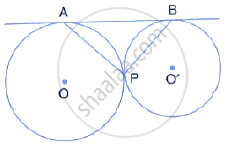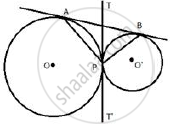Share

# In the Given Figure, Two Circles Touch Each Other Externally at Point P. Ab is the Direct Common Tangent of These Circles. Prove That: (Ii) Angles Apb = 90° - Mathematics

Course

#### Question

In the given figure, two circles touch each other externally at point P. AB is the direct common tangent of these circles. Prove that:(ii) angles APB = 90°

#### Solutionii) Now in Δ ATP ,
∴ ∠TAP = ∠TPA
Similarly in Δ BTP,∠TBP = ∠TPB
∠TAP +∠TBP =∠APB
But
∴ TAP + ∠TBP  + ∠APB =180°
⇒ ∠APB =  ∠TAP  + ∠TBP =90°

Is there an error in this question or solution?